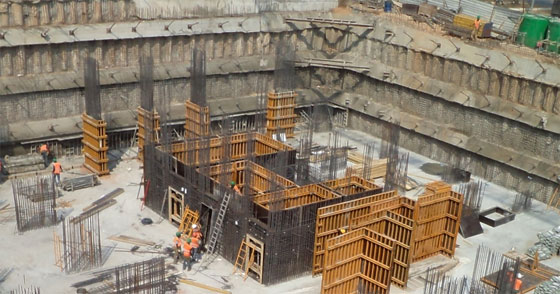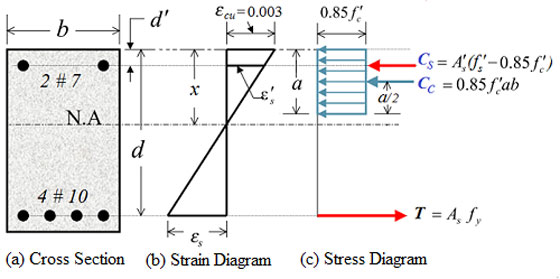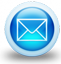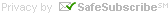# Strength of Doubly Reinforced Concrete Beam (compression steel not yielding)Some useful construction tips to calculate the nominal flexural strength Mn concerning the reinforced concrete rectangular section. It is illustrated in figure 9-3(a).

The provided section is reinforced twice with steel in tension and compression zone of the section. The guidelines of ACI-318 is followed for calculating the nominal flexural strength Mn. The highest value of applicable strain at the utmost concrete fiber is supposed to be 0.003.

For fc' grerater than 4000 psi the value of ß1 is calculated as given below;
ß1 = 0.85 - 0.05 {( fc' -4000)/1000} = 0.8
Assume that the compression steel has yielded when the strength is reached (strain in concrete is 0.003).

Given that tension steel consists of 4 bars of #10 (dia 1.27 in.).
Area of one bar of #10 = 1.27 in2.
As = 4-#10 bars. = 4 (1.27) = 5.08 in2.

Area of compression steel , As' = 2-#7 = 2(0.6) = 1.2 in2. The internal forces acting on the section shown in figure 9-3(c) are calculated as given below;
Cc = 0.85 fc' ba = 0.85 (5) (14) a = 59.5 a
Cs = (fs' - 0.85 fc') As' = (60 - 0.85*5) 1.2 = 66.9 kips
T = As fy = (5.08) (60) = 304.8 kips

Applying static equilibrium, we get Cc + Cs = T;
59.5 a + 66.9 = 304.8
therefore depth of stress block, a = (304.8- 66.9)/59.5 = 3.998 in Depth of neutral axis x = a / ß1 = 3.998/0.8 = 4.997 in# Takeoff Tools